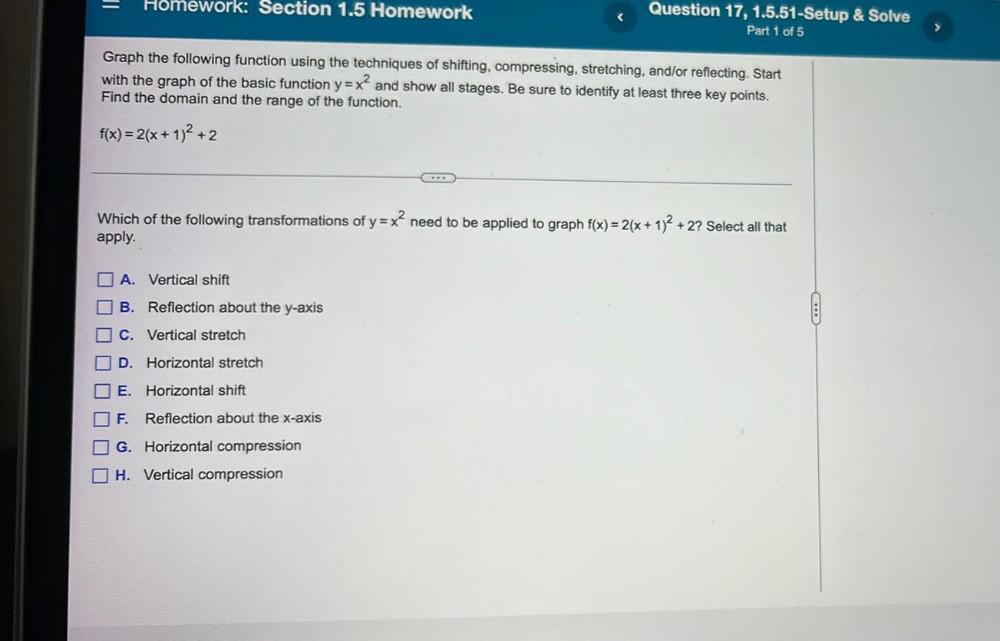Question:

# Homework: Section 1.5 Homework Question 17, 1.5.51-Setup & Solve Part 1 of 5 Graph the following function using the techniques oHomework: Section 1.5 Homework Question 17, 1.5.51-Setup & Solve Part 1 of 5 Graph the following function using the techniques of shifting, compressing, stretching, and/or reflecting. Start with the graph of the basic function y=x? and show all stages. Be sure to identify at least three key points. Find the domain and the range of the function. f(x)=2(x + 1)2 + 2 Which of the following transformations of y=x? need to be applied to graph f(x) = 2(x + 1)2 + 2? Select all that apply. 2 O A. Vertical shift B. Reflection about the y-axis C. Vertical stretch O D. Horizontal stretch E. Horizontal shift F. Reflection about the x-axis I G. Horizontal compression H. Vertical compression